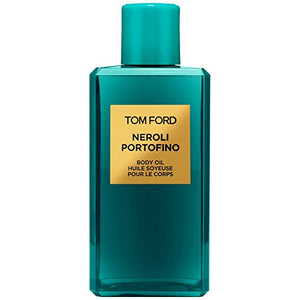# Tom Ford Neroli Portofino Body Oil 250Ml

• \$277.48

• P

• l

• e

• a

• s

• e

• b

• e

• a

• w

• a

• r

• e

• t

• h

• a

• t

• u

• n

• l

• e

• s

• s

• e

• x

• p

• r

• e

• s

• s

• l

• y

• i

• n

• d

• i

• c

• a

• t

• e

• d

• o

• t

• h

• e

• r

• w

• i

• s

• e

• ,

• C

• o

• o

• k

• i

• n

• g

• M

• a

• r

• v

• e

• l

• l

• o

• u

• s

• a

• r

• e

• n

• o

• t

• t

• h

• e

• t

• h

• e

• m

• a

• n

• u

• f

• a

• c

• t

• u

• r

• e

• r

• o

• f

• t

• h

• i

• s

• p

• r

• o

• d

• u

• c

• t

• .

• P

• r

• o

• d

• u

• c

• t

• p

• a

• c

• k

• a

• g

• i

• n

• g

• m

• a

• y

• v

• a

• r

• y

• f

• r

• o

• m

• w

• h

• a

• t

• i

• s

• s

• h

• o

• w

• n

• o

• n

• t

• h

• i

• s

• l

• i

• s

• t

• i

• n

• g

• .

• W

• e

• r

• e

• c

• o

• m

• m

• e

• n

• d

• t

• h

• a

• t

• y

• o

• u

• d

• o

• n

• o

• t

• r

• e

• l

• y

• s

• o

• l

• e

• l

• y

• o

• n

• t

• h

• e

• i

• n

• f

• o

• r

• m

• a

• t

• i

• o

• n

• p

• r

• e

• s

• e

• n

• t

• e

• d

• o

• n

• o

• u

• r

• l

• i

• s

• t

• i

• n

• g

• .

• P

• l

• e

• a

• s

• e

• a

• l

• w

• a

• y

• s

• r

• e

• a

• d

• t

• h

• e

• l

• a

• b

• e

• l

• s

• ,

• w

• a

• r

• n

• i

• n

• g

• a

• n

• d

• d

• i

• r

• e

• c

• t

• i

• o

• n

• s

• p

• r

• o

• v

• i

• d

• e

• d

• b

• e

• f

• o

• r

• e

• u

• s

• i

• n

• g

• o

• r

• c

• o

• n

• s

• u

• m

• i

• n

• g

• t

• h

• e

• p

• r

• o

• d

• u

• c

• t

• .

Enter the world of Portofino, a resort town on the Italian Riviera, known for its arresting beauty and stunning old-world glamour. Inspired by the dramatic landscape and jet-set sophistication of Portofino. The Neroli Portofino scent, which is one of the most popular scents in the Private Blend Collection, has a crisp and effervescent qualiy that is entirely present element of these body products, says Tom Ford. Incredibly luxurious and silky, this lightweight revitalizing oil helps condition the skin with the signature Meditarranean based blend of olive fruit oil, grape seed oil and date seed extract. Gently scented with Tunisian neroli, Italian bergamot, sicilian lemon, winter yellow mandarin, lavender, orange flower, rosemary and amber. 250ml

every pages